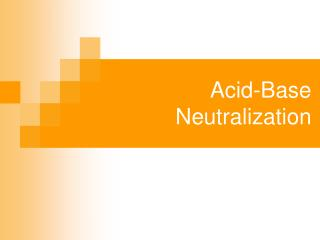DownloadDownload PresentationAcid-Base Neutralization

# Acid-Base Neutralization

Télécharger la présentation## Acid-Base Neutralization

- - - - - - - - - - - - - - - - - - - - - - - - - - - E N D - - - - - - - - - - - - - - - - - - - - - - - - - - -
##### Presentation Transcript

1. Acid-Base Neutralization

2. What is a Neutralization Reaction? • The reaction between an acid and a base produces an ionic compound (a salt) and water. This is a double displacement reaction. Acid + Base A Salt + Water HCl(aq)+ KOH(aq) KCl(aq)+ H2O(l)

3. What is a salt? • A salt is an ionic compound. • In a Neutralization Reaction, the salt is formed from the cation from the base and the anion from the acid. HNO3(aq) + NaOH(aq) NaNO3(aq) + H2O(l) The net ionic reaction for a neutralization:

4. Why is it called a Neutralization Reaction? • An acid is corrosive, and has a pH below 7. • A base is corrosive and has a pH above 7. When a base is added to an acid, in the correct proportions, the resulting solution: • is no longer corrosive, • has a pH of 7 (neutral) In short, Acid and Base properties are neutralized.

5. What does “combined in the proper proportions” mean? • In this Neutralization Reaction: 1HNO3(aq) + 1NaOH(aq) 1NaNO3(aq) + 1H2O(l) The coefficients show that 1 mole of nitric acid reacts with 1 mole of sodium hydroxide to form 1 mole of the salt, sodium nitrate and 1 mole of water. General rule about Neutralization Reactions When any strong acid reacts with any strong base in the mole ratio from the balanced chemical equation, a neutral aqueous solution of a salt is formed.

6. Practice the idea (Hey, look, Stoichiometry!): • Write the balanced neutralization equation for the reaction of sulphuric acid with potassium hydroxide: __H2SO4(aq) + __KOH(aq) __ +__ • Predict the amounts of acid or base needed to neutralize the given amounts:

7. What is the “equivalence point”? • The equivalence point is the point in the neutralization reaction when the moles of the H+ are equal to the moles of OH-. • In a neutralization of a strong acid with a strong base, the pH at the equivalence point is 7. • In a neutralization between acids and bases of different strengths (ie, weak acid, strong base or other), the pH at the equivalence point is not 7.

8. How do you know the equivalence point has been reached? • You use a pH indicator in solution form, pH paper or a pH meter. • Indicators turn different colours in different pH ranges. • For example Phenophthalein (phth) is clear and colourless in acid, in a solution around pH 7.6 it turns bright pink, so it is pink in a basic solution.

9. More Neutralization Stoichiometry • What volume of 0.575M HCl will neutralize 4.22g of solid Mg(OH)2? • Balanced chemical reaction equation: • Moles of given quantity: • Moles of unknown quantity: • Desired quantity of unknown: